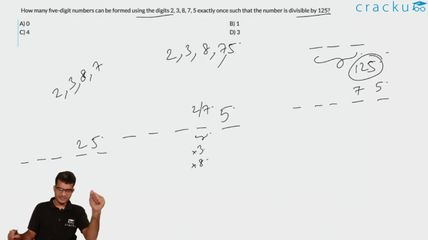Question 70

# How many five-digit numbers can be formed using the digits 2, 3, 8, 7, 5 exactly once such that the number is divisible by 125?

Solution

As we know for a number to be divisible by 125, its last three digits should be divisible by 125
So for a five digit number, with digits 2,3,8,7,5 its last three digits should be 875 and 375
Hence only 4 numbers are possible with its three digits as 875 and 375
I.e. 23875, 32875, 28375, 82375

### View Video Solution